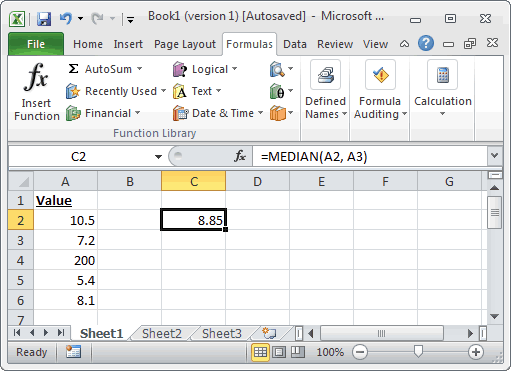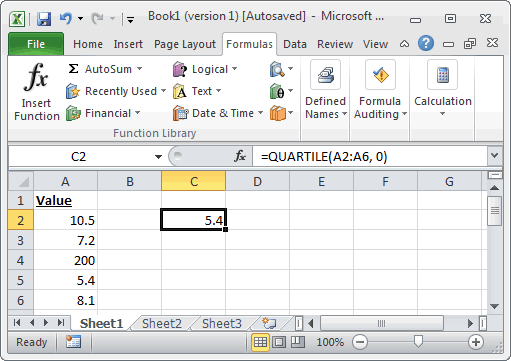# Mean

Use the “Average” Function

# Median

In Microsoft Excel, the MEDIAN function returns the median of the numbers provided.
MEDIAN( number1, [number2, … number_n] )Based on the Excel spreadsheet above, the MEDIAN function would return the following:
=MEDIAN(A2, A3)     would return 8.85
=MEDIAN(A3, A5, 45)     would return 7.2
=MEDIAN(A2:A6)     would return 8.1

# Quartile

In Microsoft Excel, the QUARTILE function returns the quartile from a set of values.
Syntax

QUARTILE( array, nth_quartile )

array is a range or array from which you want to return the nth quartile.

nth_quartile is the quartile value that you wish to return. It can be one of the following values:
Value     Explanation
0     Smallest value in the data set
1     First quartile (25th percentile)
2     Second quartile (50th percentile)
3     Third quartile (75th percentile)
4     Largest value in the data set
Note

If nth_quartile is not an integer, it will be truncated.Based on the Excel spreadsheet above, the QUARTILE function would return the following:
=QUARTILE(A2:A6, 0)     would return 5.4
=QUARTILE(A2:A6, 1)     would return 7.2
=QUARTILE(A2:A6, 2)     would return 8.1
=QUARTILE(A2:A6, 3)     would return 10.5
=QUARTILE(A2:A6, 4)     would return 200
=QUARTILE({7,8,9,10}, 0)     would return 7
=QUARTILE({7,8,9,10}, 1)     would return 7.75
=QUARTILE({7,8,9,10}, 2)     would return 8.5
=QUARTILE({7,8,9,10}, 3)     would return 9.25
=QUARTILE({7,8,9,10}, 4)     would return 10